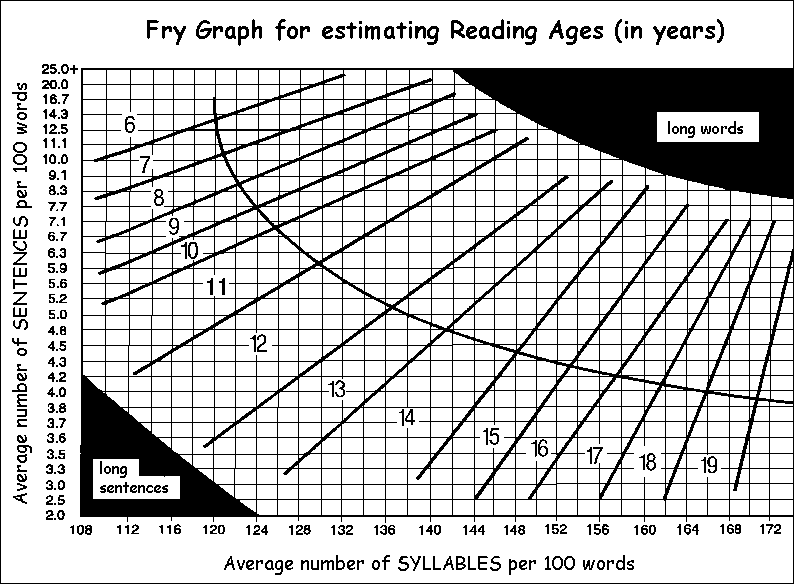# Readability of Texts

Sample text for determining readability index

Flesch-Kincaid Formula
This is a US Government Department of Defense standard test
(i) Calculate L, the average sentence length (number of words ÷ number of sentences).  Estimate the number of sentences to the nearest tenth,where necessary.
(ii) Calculate N, the average number of syllables per word (number of syllables ÷ number of words).
Then grade level = (L+N)x0.4
Reading Age = [(L+N)x0.4]+5 years

'SMOG' Formula
Select samples of 30 consecutive sentences.
In each sample, count the number of words with 3 or more syllables.
Find the average number, N.
Then grade level = (square root of N) + 3.
Reading Age = (square root of N) + 8 years.
This test tends to give higher values than the other formula, because it is intended to predict the level necessary for 100% comprehension of the text.

Fry Readability Graph
Select samples of 100 words.
(i) Find y, the average number of sentences per 100-word passage (calculating to the nearest tenth).
(ii) Find x, the average number of syllables per 100-word sample.
Then use the Fry graph (below) to determine the reading age, in years.
This test is suitable for all ages, from infant to upper secondary.
The curve represents normal texts.  Points below the curve imply longer than average sentence lengths.  Points above the curve represent text with a more difficult vocabulary.